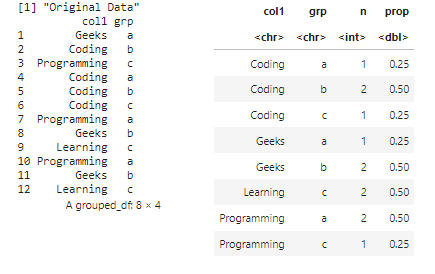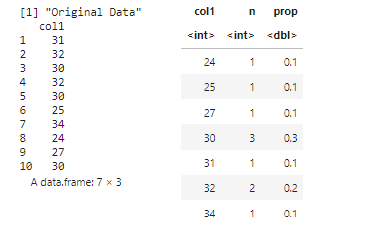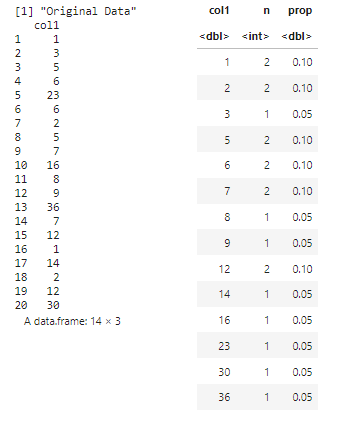Open in App
Not now

# How to Use dplyr to Generate a Frequency Table in R

• Last Updated : 09 Oct, 2022

The frequency table in R is used to create a table with a respective count for both the discrete values and the grouped intervals. It indicates the counts of each segment of the table. It is helpful for constructing the probabilities and drawing an idea about the data distribution.

The dplyr package is used to perform simulations in the data by performing manipulations and transformations. It can be installed into the working space using the following command :

`install.packages("dplyr")`

A data frame in R can be created using the data.frame() method in R . The frequency table can be constructed by using the count() method appended to the pipe operator of the data frame.

Syntax : count(df-cols)

### Create Frequency Table by Group

The groups are chosen as unique sets from the columns that are specified in the count() method. The columns in the output are all the input columns of the count() method.

The group_by method can also be appended to using the pipe operator to group the data by the specified column. The probabilities and the count can also be returned using the mutate() method. The probabilities can be specified using the prop.table(n), which also uses the count of the values in the data frame column.

## R

 `library``(dplyr)`` ` `# creating a data frame ``data_frame <- ``data.frame``(col1 = ``c``(``"Geeks"``,``"Coding"``,``                                  ``"Programming"``,``"Coding"``,``                                  ``"Coding"``,``"Coding"``,``                                  ``"Programming"``,``"Geeks"``,``                                  ``"Learning"``,``"Programming"``,``                                  ``"Geeks"``,``"Learning"` `),``                         ``grp = ``c``(``rep``(``c``(``"a"``,``"b"``,``"c"``),4)))``print``(``"Original Data"``)``print``(data_frame)`` ` `# creating a frequency table using col1 ``# and grp columns of data frame``data_frame %>%``  ``count``(col1, grp) %>%`` ` `  ``# now required with changes to dplyr::count()``  ``group_by``(grp) %>%          ``  ``mutate``(prop = ``prop.table``(n))`

Output### Using rpois() method

Functions can also be used to generate the vector of integers or string values. The rpois() method in R is used to draw randomly computed poisson density.

Syntax : rpois(num-of-observations, rate=rate )

The counts and their respective probabilities can be calculated using the count() and mutate() methods. The prop.table can be used to compute probabilities.

## R

 `library``(``"dplyr"``)`` ` `# using a func to generate values``vec <- ``rpois``(10,30)`` ` `# creating a data frame ``data_frame <- ``data.frame``(col1 = vec)``print``(``"Original Data"``)``print``(data_frame)`` ` `# creating a frequency table using col1 columns of data frame``data_frame %>%`` ` `  ``# now required with changes to dplyr::count()``  ``count``(col1) %>%        ``  ``mutate``(prop = ``prop.table``(n))`

Output### By compute the probability

The frequency can be calculated using the dplyr package in R by specifying the vector in the count() method. The respective counts are computed along with the segments into which the integral values fall. The prop.table is used to compute the probabilities of the computed counts.

## R

 `library``(``"dplyr"``)`` ` `# using a func to generate values``# creating a data frame ``data_frame <- ``data.frame``(col1 = ``c``(1,3,5,6,23,6,2,5,7,16,8,``                                  ``9,36,7,12,1,14,2,12,30))``print``(``"Original Data"``)``print``(data_frame)`` ` `# creating a frequency table using col1 columns of data frame``data_frame %>%`` ` `  ``# now required with changes to dplyr::count()``  ``count``(col1) %>%        ``  ``mutate``(prop = ``prop.table``(n))`

OutputMy Personal Notes arrow_drop_up